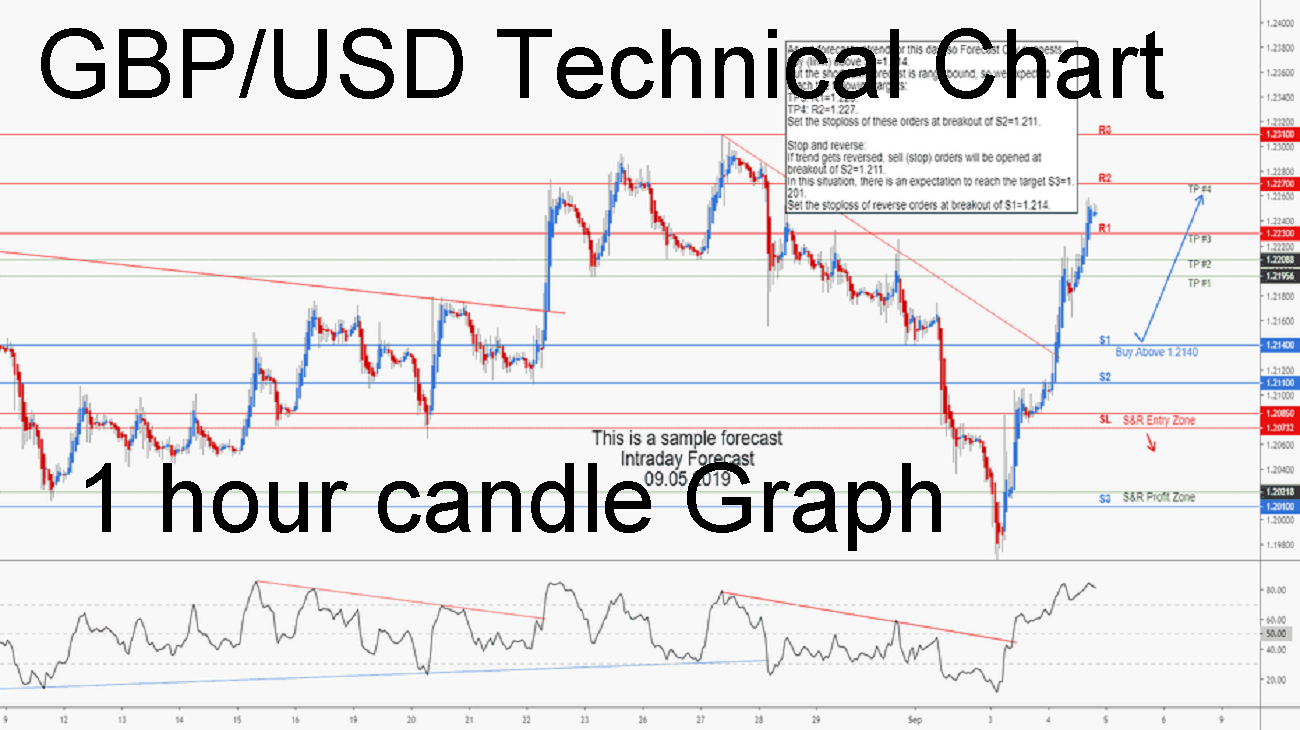As we forecast uptrend for this day, so Forecast City suggests buy (limit) above S1=1.214.
But the short term forecast is range bound, so we expect to reach the following targets:
TP3: R1=1.223.
TP4: R2=1.227.
Set the stoploss of these orders at breakout of S2=1.211.

Stop and reverse:
If trend gets reversed, sell (stop) orders will be opened at breakout of S2=1.211.
In this situation, there is an expectation to reach the target S3=1.201.
Set the stoploss of reverse orders at breakout of S1=1.214.

Donate

ETH (ERC20) : 0xe1f1b958189f510df220b338d82f555193b07316